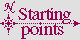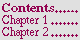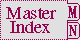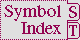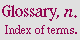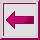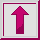V

valid array dimension n. a fixnum suitable for use as an array dimension. Such a fixnum must be greater than or equal to zero, and less than the value of array-dimension-limit. When multiple array dimensions are to be used together to specify a multi-dimensional array, there is also an implied constraint that the product of all of the dimensions be less than the value of array-total-size-limit.

valid array index n. (of an array) a fixnum suitable for use as one of possibly several indices needed to name an element of the array according to a multi-dimensional Cartesian coordinate system. Such a fixnum must be greater than or equal to zero, and must be less than the corresponding dimension of the array. (Unless otherwise explicitly specified, the phrase ``a list of valid array indices'' further implies that the length of the list must be the same as the rank of the array.) ``For a 2 by 3 array, valid array indices for the first dimension are 0 and 1, and valid array indices for the second dimension are 0, 1 and 2.''

valid array row-major index n. (of an array, which might have any number of dimensions) a single fixnum suitable for use in naming any element of the array, by viewing the array's storage as a linear series of elements in row-major order. Such a fixnum must be greater than or equal to zero, and less than the array total size of the array.

valid fill pointer n. (of an array) a fixnum suitable for use as a fill pointer for the array. Such a fixnum must be greater than or equal to zero, and less than or equal to the array total size of the array.

valid logical pathname host n. a string that has been defined as the name of a logical host. See the function load-logical-pathname-translations.

valid pathname device n. a string, nil, :unspecific, or some other object defined by the implementation to be a valid pathname device.

valid pathname directory n. a string, a list of strings, nil, :wild, :unspecific, or some other object defined by the implementation to be a valid directory component.

valid pathname name n. a string, nil, :wild, :unspecific, or some other object defined by the implementation to be a valid pathname name.

valid pathname type n. a string, nil, :wild, :unspecific.

valid pathname version n. a non-negative integer, or one of :wild, :newest, :unspecific, or nil. The symbols :oldest, :previous, and :installed are semi-standard special version symbols.

valid physical pathname host n. any of a string, a list of strings, or the symbol :unspecific, that is recognized by the implementation as the name of a host.

valid sequence index n. (of a sequence) an integer suitable for use to name an element of the sequence. Such an integer must be greater than or equal to zero, and must be less than the length of the sequence. (If the sequence is an array, the valid sequence index is further constrained to be a fixnum.)

value n. 1. a. one of possibly several objects that are the result of an evaluation. b. (in a situation where exactly one value is expected from the evaluation of a form) the primary value returned by the form. c. (of forms in an implicit progn) one of possibly several objects that result from the evaluation of the last form, or nil if there are no forms. 2. an object associated with a name in a binding. 3. (of a symbol) the value of the dynamic variable named by that symbol. 4. an object associated with a key in an association list, a property list, or a hash table.

value cell n. Trad. (of a symbol) The place which holds the value, if any, of the dynamic variable named by that symbol, and which is accessed by symbol-value. See cell.

variable n. a binding in the ``variable'' namespace. See Section 3.1.2.1.1 (Symbols as Forms).

vector n. a one-dimensional array.

vertical-bar n. the standard character that is called ``vertical bar'' (|). See Figure 2-5.

The following X3J13 cleanup issues, not part of the specification, apply to this section: